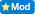The sequence of the Primes [prime numbers][

I have just written an account of how this problem might be resolved. This relates to the distibution rules in elementary [school algebra].the account saved on the computer? This is my question. William P. G. Shaw. e-mail address: wpgshaw@hotmail.com

0  Views: 1176 Answers: 2 Posted: 12 years ago
Tags:

Homework here >>> http://nrich.maths.org/6413

There is a line of research into the prime sequence that has not yet, to my knowledge, been done so thus far.

An arithmetical result is that an uneven composite integer having two integer factors can be expressed as the difference of two squares of integers, one of the squares being even, the other square uneven. The prime factors are then the two integers that have been squared. If these two squared numbers can be found then the factors are known. It is very easy to make up examples of this.

In an analogous manner, if a prime number can be expressed as the sum of two squares, then this immediately factors into a complex number and its conjugate, where the real and imaginary parts are integers. If this can be done for a series of prime numbers then it may just transpire that the double series of real and imaginary parts may be predictable, and the successor primes directly computed. Elementary logic and Diophantine equations might be involved. For example, an algebraic expression has to represent an even integer if there is an even integer on the other side of the equality sign.

The sequence of the primes has remained an unsolved problem since Euclid C. 2500BC so the student should not feel downhearted if he or she has not managed to resolve this perplexity. According to Georg F. B. Riemann, the eminent 19th century German mathematician, the prime sequence is a matter which the human mind will never be able to understand .

Never the less, the expression of primes as the sums of two integer squares looks promising.

This is not an answer, but a pointer to research, rather than hopeless speculation.

[A foot note] The sequence of primes is conventionally written as: [2, 3, 5, 7, 11, ... ] This is not really logical because the number two  functions in arithmetic very differently from the infinitude of the remaining primes: [3, 5, 7, 11, 13, ... ] It might be better to write: , [3, 5, 7, 11 ...] so placing two in a class of its own.]

WILLIAM P G SHAW

Research into prime numbers is far beyond the homework stage.
ColleenThis is a question and answer forum. Try creating a blog. We are not a blog site.

### Top contributors in Uncategorized category

ROMOS
Karma: 1101K

Colleen
Karma: 953K

country bumpkin
Karma: 838K

Benthere